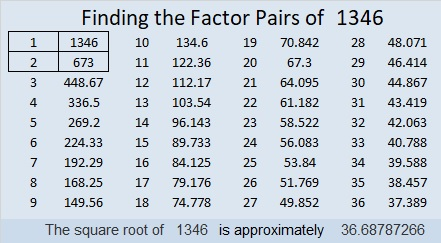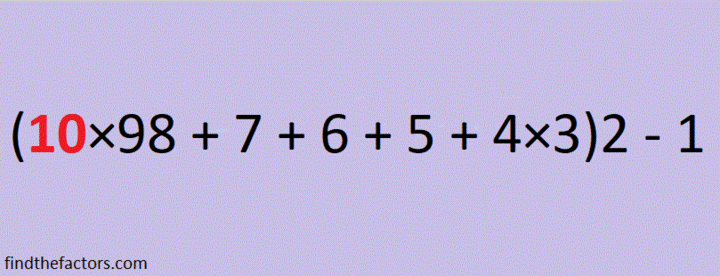(1346÷2)×3 = 2019 Magic or Square?

I was inspired to make a 3 × 3 Magic Square where every number is different but the numbers in each row, column and diagonal added together equal the same number, 54:I made it by taking a regular 3 × 3 Magic Square and adding 13 to each of its numbers.

What inspired me to do that? This magical tweet of a palindromic Magic Square for the Year 2019:

Yeah, I know my magic square isn’t quite as impressive. It might be more square than it is magic. It’s also less impressive than this number 2019 spelled out using fifty-one
4 × 4 Magic Squares.

Nevertheless, I made it, and I’m sharing it in this post I’ve numbered 1346, a special number this year because (1346÷2)×3 = 2019

Here’s more about the number 1346:

• 1346 is a composite number.
• Prime factorization: 1346 = 2 × 673
• 1346 has no exponents greater than 1 in its prime factorization, so √1346 cannot be simplified.
• The exponents in the prime factorization are 1, and 1. Adding one to each exponent and multiplying we get (1 + 1)(1 + 1) = 2 × 2 = 4. Therefore 1346 has exactly 4 factors.
• The factors of 1346 are outlined with their factor pair partners in the graphic below.1346 is the hypotenuse of a Pythagorean triple:
770-1104-1346 which is 2 times 385-552-673

Facts about and Factors of 2019

Here’s a countdown you can use to ring in the New Year:make science GIFs like this at MakeaGif

2019 is the sum of consecutive numbers three different ways:
1009 + 1010 = 2019
672 + 673 + 674 = 2019
334 + 335 + 336 + 337 + 338 + 339 = 2019

There is one way that 2019 is the sum of consecutive odd numbers:
671 + 673 + 675 = 2019

2019 is the difference of two squares two different ways:
338² – 335² = 2019
1010² – 1009² = 2019

2019 is the sum of three squares nine different ways:
43² + 13² + 1² = 2019
43² + 11² + 7² = 2019
41² + 17² + 7² = 2019
41² + 13² + 13² = 2019
37² + 25² + 5² = 2019
37² + 23² + 11² = 2019
37² + 19² + 17² = 2019
35² + 25² + 13² = 2019
31² + 23² + 23² = 2019

2019 is the hypotenuse of a Pythagorean triple:
1155-1656-2019 so 1155² + 1656² = 2019²

2¹⁰ + 2⁹ + 2⁸ + 2⁷ + 2⁶ + 2⁵ + 2¹ + 2⁰ = 2019

2019 is a palindrome in a couple of bases:
It’s 5B5 in BASE 19 (B is 11 base 10) because 5(19²) + 11(19) + 5(1) = 2019,
and 3C3 in BASE 24 (C is 12 base 10) because 3(24²) + 12(24) + 3(1) = 2019

Every year has factors that often catch people by surprise. Today I would like to give you my predictions for the factors of 2019:
2019 will have four positive factors: 1, 3, 673, and 2019
However, 2019 will also have four negative factors: -1, -3, -673, and -2019

Which factors, positive or negative, will be your focus in the coming year?